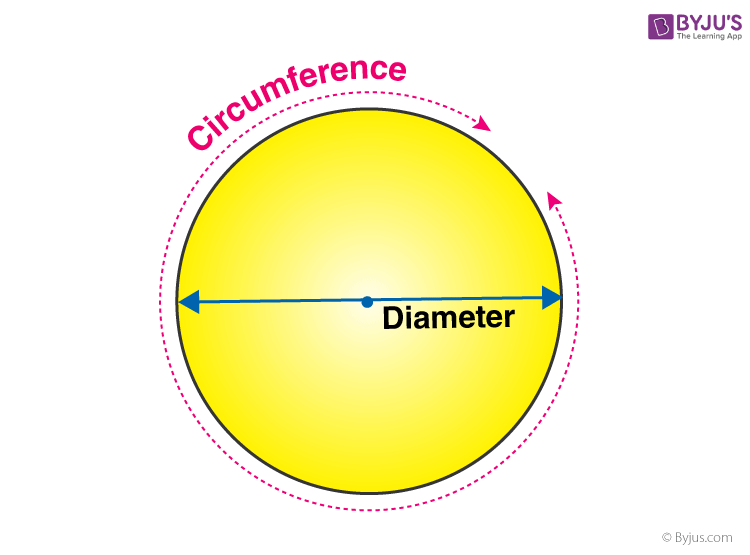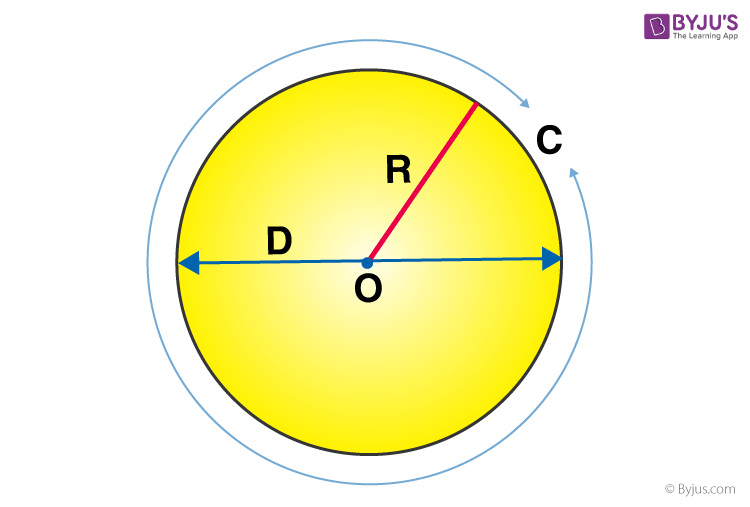# Circumference of a Circle

Circumference of the circle or perimeter of the circle is the measurement of the boundary of the circle. Whereas the area of circle defines the region occupied by it.  If we open a circle and make a straight line out of it, then its length is the circumference. It is usually measured in units, such as cm or unit m.

When we use the formula to calculate the circumference of the circle, then the radius of the circle is taken into account. Hence, we need to know the value of the radius or the diameter to evaluate the perimeter of the circle.

## Circumference of a Circle Formula

The Circumference (or) perimeter of a circle = 2πR

where,

R is the radius of the circle

π is the mathematical constant with an approximate (up to two decimal points) value of 3.14

Again,

Pi (π) is a special mathematical constant; it is the ratio of circumference to diameter of any circle.

where C = π D

C is the circumference of the circle

D is the diameter of the circle

For example: If the radius of the circle is 4cm then find its circumference.

Circumference = 2πr

= 2 x 3.14 x 4

= 25.12 cm

Also, check:

### Area of a Circle Formula

Area of any circle is the region enclosed by the circle itself or the space covered by the circle. The formula to find the area of the circle is;

A = πr2

Where r is the radius of the circle, this formula is applicable to all the circles with different radii.

## Perimeter of Semi-Circle

The semi-circle is formed when we divide the circle into two equal parts. Therefore, the perimeter of the semi-circle also becomes half.

Hence, Perimeter = 2πr/2 = πr

## Area of Semi-Circle

Area of the semi-circle is the region occupied by a semi-circle in a 2D plane. The area of the semi-circle is equal to half of the area of a circle, whose radii are equal.

Therefore, Area = πr2/2

Summary

 Circumference of Circle 2πr Area of circle πr2 Perimeter of semi-circle πr Area of semi-circle πr2/2

The distance from the centre to the outer line of the circle is called a radius. It is the most important quantity of the circle based on which formulas for area and circumference of the circle are derived. Twice the radius of a circle is called the diameter of the circle. The diameter cuts the circle into two equal parts, which is called a semi-circle.

## What is the Circumference of Circle?

The circumference is the distance around a circle or any curved geometrical shape. It is the one-dimensional linear measurement of the boundary across any two-dimensional circular surface. It follows the same principle behind finding the perimeter of any polygon, which is why calculating the circumference of a circle which is also known as the perimeter of a circle.

A circle is defined as a shape with all the points are equidistant from a point at the centre. The circle depicted below has its centre lies at point A.

The value of pi is approximately 3.1415926535897… and we use a Greek letter π (pronounced as Pi) to describe this number. The value π is a non-terminating value.

For circle A (as given below), the circumference and the diameter will be-In other words, the distance surrounding a circle is known as the circumference of the circle. The diameter is the distance across a circle through the centre, and it touches the two points of the circle perimeter. π shows the ratio of the perimeter of a circle to the diameter. Therefore, when you divide the circumference by the diameter for any circle, you obtain a value close enough to π. This relationship can be explained by the formula mentioned below.

C/d = π

Where C indicates circumference and d indicates diameter. A different way to put up this formula is C = π × d. This formula is mostly used when the diameter is mentioned, and the perimeter of a circle needs to be calculated.

## How to Find Circumference?

Method 1: Since it is a curved surface, we can’t physically measure the length of a circle using a scale or ruler. But this can be done for polygons like squares, triangles and rectangles. Instead, we can measure the circumference of a circle using a thread. Trace the path of the circle using the thread and mark the points on the thread. This length can be measured using a normal ruler.

Method 2: An accurate way of knowing the circumference of a circle is to calculate it. For this, the radius of the circle has to be known. The radius of a circle is the distance from the centre of the circle and any point on the circle itself. The figure below shows a circle with radius R and centre O. The diameter is twice the radius of the circle.## Solved Examples

Question 1: What is the circumference of the circle with diameter 4 cm?

Solution:

Since the diameter is known to us, we can calculate the radius of the circle,

Therefore, Circumference of the Circle = 2 x 3.14 x 2 = 12.56 cm.

Question 2: Find the radius of the circle having C =  50 cm.

Solution:

Circumference = 50 cm

As per formula,  C = 2 π  r

This implies, 50 = 2 π  r

50/2 = 2 π  r/2

25 = π  r

or r =  25/π

Therefore, the radius of the circle is 25/π  cm.

### Practise Questions

1. Calculate the perimeter of a circle whose diameter is 8 cm.
2. What will be the diameter of a circle if it’s C =  10 cm?
3. If C =  12 cm, what will be its radius?
4. What is the circumference of a 16-inch circle?
5. What is the circumference of a 6 mm circle?

To learn all concepts in Math in a more engaging way, register at BYJU’S. Also, watch interesting videos on various Maths topics by downloading BYJU’S– The Learning App from Google Play Store or the app store.

### What is the Circumference of a Circle?

The circumference of a circle is defined as the linear distance around it. In other words, if a circle is opened to form a straight line then the length of that line will be the circle’s circumference.

### How to Calculate the Circumference of a Circle?

To calculate the circumference of a circle, multiply the diameter of the circle with π (pi). The circumference can also be calculated by multiplying 2×radius with pi (π=3.14).

### How to Calculate Diameter from Circumference?

The formula for circumference = diameter × π

Or, diameter = circumference/π

So, the diameter of the circle in terms of circumference will be equal to the ratio of the circumference of the circle and pi.

### What is the Circumference of a Circle with Radius 24 inches?

Circumference = 2×π×r

C = 2×3.14×24

C = 150.72 inches